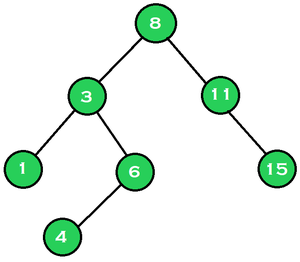Related Articles
Median of all nodes from a given range in a Binary Search Tree ( BST )
• Difficulty Level : Medium
• Last Updated : 03 May, 2021

Given a Binary Search Tree (BST)consisting of N nodes and two nodes A and B, the task is to find the median of all the nodes in the given BST whose values lie over the range [A, B].

Examples:

Input: A = 3, B = 11Output: 6
Explanation:
The nodes that lie over the range [3, 11] are {3, 4, 6, 8, 11}. The median of the given nodes is 6.

Input: A = 6, B = 15Output: 9.5

Approach: The given problem can be solved by performing any tree traversal on the given tree and store all the nodes lies over the range [A, B], and find the median of all the stored element. Follow the steps below to solve the problem:

Below is the implementation of the above approach:

## C++

 `// C++ program for the above approach ` ` `  `#include ` `using` `namespace` `std; ` ` `  `// Tree Node structure ` `struct` `Node { ` `    ``struct` `Node *left, *right; ` `    ``int` `key; ` `}; ` ` `  `// Function to create a new BST node ` `Node* newNode(``int` `key) ` `{ ` `    ``Node* temp = ``new` `Node; ` `    ``temp->key = key; ` `    ``temp->left = temp->right = NULL; ` `    ``return` `temp; ` `} ` ` `  `// Function to insert a new node with ` `// given key in BST ` `Node* insertNode(Node* node, ``int` `key) ` `{ ` `    ``// If the tree is empty, ` `    ``// return a new node ` `    ``if` `(node == NULL) ` `        ``return` `newNode(key); ` ` `  `    ``// Otherwise, recur down the tree ` `    ``if` `(key < node->key) ` `        ``node->left = insertNode( ` `            ``node->left, key); ` ` `  `    ``else` `if` `(key > node->key) ` `        ``node->right = insertNode( ` `            ``node->right, key); ` ` `  `    ``// Return the node pointer ` `    ``return` `node; ` `} ` ` `  `// Function to find all the nodes that ` `// lies over the range [node1, node2] ` `void` `getIntermediateNodes( ` `    ``Node* root, vector<``int``>& interNodes, ` `    ``int` `node1, ``int` `node2) ` `{ ` `    ``// If the tree is empty, return ` `    ``if` `(root == NULL) ` `        ``return``; ` ` `  `    ``// Traverse for the left subtree ` `    ``getIntermediateNodes(root->left, ` `                         ``interNodes, ` `                         ``node1, node2); ` ` `  `    ``// If a second node is found, ` `    ``// then update the flag as false ` `    ``if` `(root->key <= node2 ` `        ``and root->key >= node1) { ` `        ``interNodes.push_back(root->key); ` `    ``} ` ` `  `    ``// Traverse the right subtree ` `    ``getIntermediateNodes(root->right, ` `                         ``interNodes, ` `                         ``node1, node2); ` `} ` ` `  `// Function to find the median of all ` `// the values in the given BST that ` `// lies over the range [node1, node2] ` `float` `findMedian(Node* root, ``int` `node1, ` `                 ``int` `node2) ` `{ ` `    ``// Stores all the nodes in ` `    ``// the range [node1, node2] ` `    ``vector<``int``> interNodes; ` ` `  `    ``getIntermediateNodes(root, interNodes, ` `                         ``node1, node2); ` ` `  `    ``// Store the size of the array ` `    ``int` `nSize = interNodes.size(); ` ` `  `    ``// Print the median of array ` `    ``// based on the size of array ` `    ``return` `(nSize % 2 == 1) ` `               ``? (``float``)interNodes[nSize / 2] ` `               ``: (``float``)(interNodes[(nSize - 1) / 2] ` `                         ``+ interNodes[nSize / 2]) ` `                     ``/ 2; ` `} ` ` `  `// Driver Code ` `int` `main() ` `{ ` `    ``// Given BST ` `    ``struct` `Node* root = NULL; ` `    ``root = insertNode(root, 8); ` `    ``insertNode(root, 3); ` `    ``insertNode(root, 1); ` `    ``insertNode(root, 6); ` `    ``insertNode(root, 4); ` `    ``insertNode(root, 11); ` `    ``insertNode(root, 15); ` ` `  `    ``cout << findMedian(root, 3, 11); ` ` `  `    ``return` `0; ` `} `

Output:

```6
```

Time Complexity: O(N)
Auxiliary Space: O(N)

Attention reader! Don’t stop learning now. Get hold of all the important mathematical concepts for competitive programming with the Essential Maths for CP Course at a student-friendly price. To complete your preparation from learning a language to DS Algo and many more,  please refer Complete Interview Preparation Course.

My Personal Notes arrow_drop_up
Recommended Articles
Page :